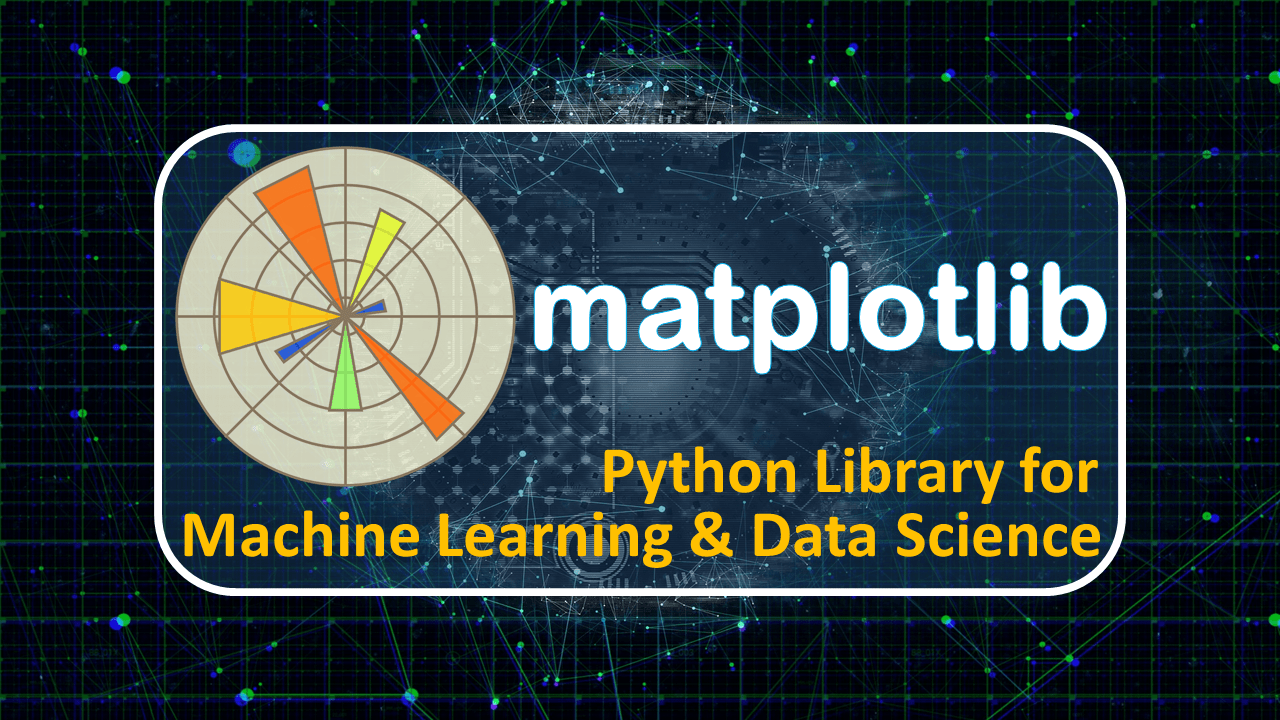## Python Matplotlib Tutorial – Mastery in Matplotlib Library

Python Matplotlib Tutorial After complication of python NumPy Tutorial and python pandas tutorial. Now, we jump on the python matplotlib tutorial to become a master in it. What is python matplotlib? Matplotlib is a 2D and 3D graph plotting python library. It also supports to create animations and images. This is an advanced part of python matplotlib.  Using python matplotlib, you can draw a...
Top